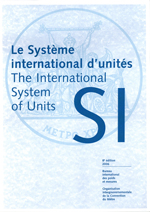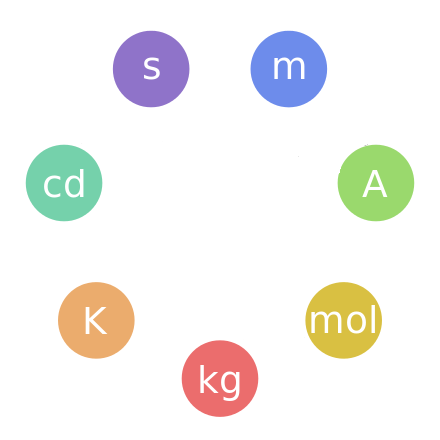# Common SI UnitsScientists use a system of units called the SI units (Système International d'Unités) that have been agreed on so that their work can be understood throughout the world. Most of the SI units are the same as the metric units of measurement, but there are a few differences. In this article, we're going to look at some of the common SI units. The units are split up into two types: base units and derived units. The derived units are built up out of the base units. There are seven SI base units and 22 derived units. There is also a set of twenty prefixes that can be assigned to multiples and fractions of SI units.

## The SI Base UnitsQuantity Unit Abbreviation
Time second s
Length metre m
Mass kilogram kg
Temperature Kelvin K
Electrical Current Ampere A
Amount of Substance mole mol
Luminous Intensity candela cd

## Some Common Derived Units

These units are built from the base units:

Quantity Unit Abbreviation
Acceleration metres per second squared $\text{m/s}^2$
Angular Acceleration radians per second squared $\text{rad/s}^2$
Area square metre $\text{m}^2$
Density kilograms per cubic metre $\text{kg/m}^3$
Energy Joule J (Nm)
Force Newton N ($\text{kgm/s}^2$)
Frequency Hertz Hz (1/s)
Impulse Newton-second kgm/s
Moment of Force Newton-metre Nm
Power Watt W (or J/s)
Pressure Pascal Pa (or $\text{N/m}^2$)
Velocity metres per second m/s
Volume (solid) cubic metres $\text{m}^3$
Volume (liquid) Litre L (or $10^{-3} \text{ m}^3$)
Work Joule J (or Nm)

### Description

• Metric numbers
• Speed
• Conversions

and several other topics

### Audience

Year 10 or higher, some chapters are suitable for Year 8+ students

### Learning Objectives

Learn about measurement and related topics

Author: Subject Coach
You must be logged in as Student to ask a Question.